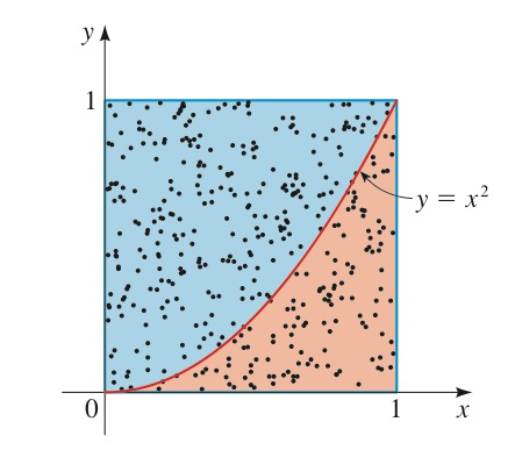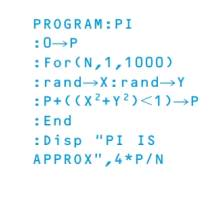Chapter 14.FOM, Problem 6P### Algebra and Trigonometry (MindTap ...

4th Edition
James Stewart + 2 others
ISBN: 9781305071742

#### Solutions

Chapter
Section### Algebra and Trigonometry (MindTap ...

4th Edition
James Stewart + 2 others
ISBN: 9781305071742
Textbook Problem

# Areas of Curved Regions The Monte Carlo method can be used to estimate the area under the graph of a function. The figure below shows the region under the graph of f ( x ) = x 2 , above the x -axis, between x = 0 and x = 1 . If we choose a point in the square at random, the probability that it lies under the graph of f ( x ) = x 2 is the area under the graph divided by the area of the square. So if we randomly select a large number of point in the square, we have number of hits under the graph number of hits in the square ≈ area under  graph area   of   square Modify the program from Problem 5 to carry out this Monte Carlo simulation and approximate the required area.To determine

The required area using Monte Carlo simulation.

Explanation

Given:

1) The circle in the figure (1) has radius 1 and the square has area 4.

2) Choose a point at random from the square, the probability that it lies under the graph will be areaunder graphareaofsquare

3) The Monte Carlo method involves choosing many points inside the square. Then, number of hits under the graphnumber of hits in the squarearea under  graphareaofsquare

Figure (1)

Approach:

Modify the following program on the TI-83 calculator to generate 1000 random points and approximate the required area

### Still sussing out bartleby?

Check out a sample textbook solution.

See a sample solution

#### The Solution to Your Study Problems

Bartleby provides explanations to thousands of textbook problems written by our experts, many with advanced degrees!

Get Started